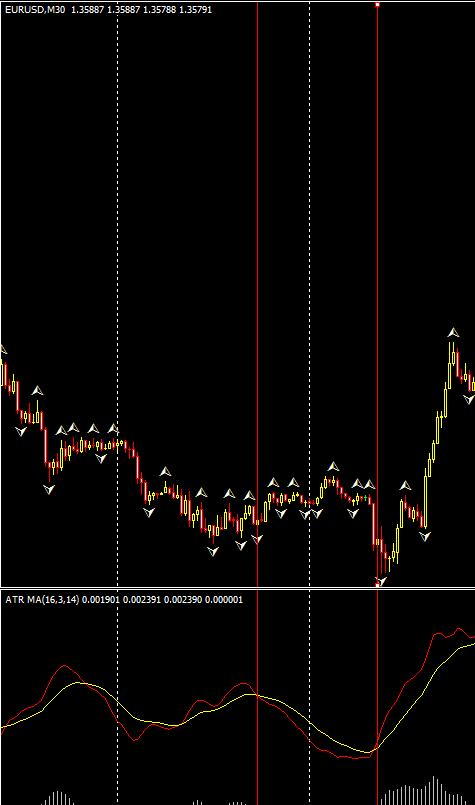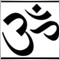# How do I get high/low of fractal values as far back as the last X indicator signal?73

Hey everyone, I haven't posted here much, but I try not to come here asking people to 'do work for me'... having said that, I would appreciate some advice as I'm a beginner with coding.

Here's my problem... I'm trying to create a fractal breakout zone around the price for as long as the ATR is under its SMA (approximately midnight to 8am), and then after the ATR moves above its SMA, I want to buy/sell when the price closes above/below the bounds of the zone. I need to code for the upper and lower value of the fractals, but only as far back as the last time the ATR crossed its MA. Is there a way to do that? I coded a custom indicator to display the ATR along with two of its moving averages, and I added comments to the code below + an image to explain it.

```double   ATRMA             = iCustom(NULL, 0, "ATR MA", ATRPeriod, BaseSMA, SignalSMA, 1, 0); // Fast MA of ATR, essentially a slightly-smoothed ATR
double   ATRSignal         = iCustom(NULL, 0, "ATR MA", ATRPeriod, BaseSMA, SignalSMA, 2, 0); // Slower MA of ATR, the 'signal line' in this case

double   ATRMA_Shift       = iCustom(NULL, 0, "ATR MA", ATRPeriod, BaseSMA, SignalSMA, 1, 1);
double   ATRSignal_Shift   = iCustom(NULL, 0, "ATR MA", ATRPeriod, BaseSMA, SignalSMA, 2, 1);

double   CloseLastBar      = iClose(NULL, 0, 1)
double   CloseTwoBars      = iClose(NULL, 0, 2)

if (ATRMA_Shift > ATRSignal_Shift && ATRMA < ATRSignal)  //Define PreviousSessionClose as the last time ATR crossed under its MA
{
datetime PreviousSessionClose = TimeCurrent();
}

if (ATRMA_Shift < ATRSignal_Shift && ATRMA > ATRSignal)  //Define SessionOpen as the last time ATR crossed above its MA
{
datetime SessionOpen = TimeCurrent();
int LowVolumeBars = NormalizeDouble((SessionOpen-PreviousSessionClose)/Period(), 1); // Get number of bars since ATR crossed under its MA

for (int k = 0; k < LowVolumeBars; k ++)
{
double UpperBreakoutRange = iFractals(NULL, 0, MODE_UPPER, k);
double LowerBreakoutRange = iFractals(NULL, 0, MODE_LOWER, k);
}
}

if (CloseTwoBars < UpperBreakoutRange && CloseLastBar > UpperBreakoutRange) Order = SIGNAL_BUY;  // Send an order with the next bar opening if the price closes outside of the breakout range from the inside of it
if (CloseTwoBars > LowerBreakoutRange && CloseLastBar < LowerBreakoutRange) Order = SIGNAL_SELL;
```So, in the above image, I want to define the breakout range as the high/low of all fractals between the two red lines. Thus, the EA would have triggered a buy order in this example about 6.5 hours (12-13 bars or so) after the ATR crossed its MA upward. Thanks again for any help you can offer, and I hope I've been clear enough!

-Alex5299

Looks like you're a stronger coder than me. However, here's my suggestion for what it's worth. Perhaps you can save the Time the ATR crossed in a Static Variable. Then check the bars with that Time and use the Fractals from then.73

Thanks for the response ubzen, but you'd be mistaken to label me as a strong coder! I am making an effort to learn, though.

Anyways, could you explain what you mean? Hopefully, this code is meant to get the time now, subtract from it the time of last ATR cross, and divide by the period to get the number of bars -- which seems to match your suggestion:

```if (ATRMA_Shift > ATRSignal_Shift && ATRMA < ATRSignal)  //Define PreviousSessionClose as the last time ATR crossed under its MA
{
static datetime PreviousSessionClose = TimeCurrent();
}

if (ATRMA_Shift < ATRSignal_Shift && ATRMA > ATRSignal)  //Define SessionOpen as the last time ATR crossed above its MA
{
static datetime SessionOpen = TimeCurrent();
int LowVolumeBars = NormalizeDouble((SessionOpen-PreviousSessionClose)/Period(), 1); // Get number of bars since ATR crossed under its MA
```

The part I don't understand, however, is saving the time in a static variable... what would that change? Do I just add "static" before the Time variable lines, as they are? See edits in red. I've never used static variables before, so I don't know the purpose behind them. Thanks for your help.5299

Global Variable also save their values like static variables and are available to other functions.

Something like this. You can replace the Macd with your indicator. I'm not sure how it's gonna work so let me know. This is intended for learning, I wouldn't run the codes below on a life real money account. A more streamline version would be to check the time and fractal using an i-loop on bars. ex) for(int i=Bars-1;i>=0;i--){if(iFractals(NULL,0,MODE_UPPER,i)>0){Return Fractal; Return Bar;}} then you can check the time of the Bar using the Bar# to see if it's > time of Crossing. Well, anyway if it was easier I would have written the below that way lol.

Btw, From what I remember about Fractals, they can be tricky, what you see on the charts is not what you get in real time. There's usually a lag of about 3 bars before it forms or something like that. Sorry I don't employ fractals or I would have had a more definite answer for you.

```//##################################################################
//##################################################################
extern double Lots=0.1;
extern int Stop_Losses=50,Take_Profit=100;
double Point2Pip,Slippage,Digit_Info,Stop_Level;
//##################################################################
//##################################################################
//----------Get_MarketInfo-Function:
int init(){
//----------Market_Info:
Digit_Info=   MarketInfo(Symbol(),MODE_DIGITS);
Stop_Level=   MarketInfo(Symbol(),MODE_STOPLEVEL);
//----------Point2Pip:
Point2Pip=Point;
if(Digit_Info==3){Point2Pip=0.01;}
if(Digit_Info==5){Point2Pip=0.0001;}
//----------
return(0);}
//##################################################################
//##################################################################
//----------UserDefined-Function: Asian_Close --> NewYork_Open:
bool Active_TZone(){bool Ans;
if(Hour()>=8 && Hour()<=16){Ans=true;}//These are for my Time
return(Ans);}//Others should input their time-range.
//##################################################################
//##################################################################
int start(){
if(Active_TZone()==true){//Only Run OrderSend During Active_TZone
//##################################################################
//##################################################################
//----------Indicator1 Macd:
double Macd_Current=iMACD(NULL,0,120,260,1,PRICE_OPEN,MODE_MAIN,0);
double Macd_Previous=iMACD(NULL,0,120,260,1,PRICE_OPEN,MODE_MAIN,1);
static datetime Up_Macd_Time;//Initialize Time Variable for Up Cross.
static datetime Dn_Macd_Time;//Initialize Time Variable for Down Cross.
//##################################################################
//##################################################################
//----------Indicator2 Fractals:
double Up_Fractal=iFractals(NULL,0,MODE_UPPER,3);
double Dn_Fractal=iFractals(NULL,0,MODE_LOWER,3);
static double Bull_Fractal;//Initialize Variable for Up Fractal.
static double Bear_Fractal;//Initialize Variable for Down Fractal.
static datetime Up_Frac_Time;
static datetime Dn_Frac_Time;
//##################################################################
//##################################################################
//----------Assign High/Low Fractal_Values:
if(Up_Fractal>0){
if(Up_Fractal>Bull_Fractal || Bull_Fractal==0){//Give me Highest
Bull_Fractal=Up_Fractal;//Bull_Fractal & Bear_Fractal starts at 0.
Up_Frac_Time=TimeCurrent();
}
}
//----------
if(Dn_Fractal>0){
if(Dn_Fractal<Bear_Fractal || Bear_Fractal==0){//Give me Lowest
Bear_Fractal=Dn_Fractal;/*For most prices Dn_Fractal would not be
Less than 0 but you still wanna change it when Dn appears*/
Dn_Frac_Time=TimeCurrent();
}
}
//##################################################################
//##################################################################
//----------Bullish/Buy Setup for Macd Crossing 0:
if(Macd_Previous<=0 && Macd_Current>0){//Crossed from Down Upward.
Up_Macd_Time=TimeCurrent();//Record time of Up Cross.
}
//----------Bearish/Sell Setup for Macd Crossing 0:
if(Macd_Previous>=0 && Macd_Current<0){//Crossed from Up Downward.
Dn_Macd_Time=TimeCurrent();//Record time of Down Cross.
}
//##################################################################
//##################################################################
//----------Primary Algorithm:
int Order_Trigger;
//----------Set Trigger for Long Orders;
if(Bull_Fractal>0){
if(Up_Frac_Time>=Up_Macd_Time){/*Fractals after Cross*/
Order_Trigger= 1;
}
}
}
//----------Set Trigger for Short Orders;
if(Bear_Fractal>0){
if(Bid<Bear_Fractal){
if(Dn_Frac_Time>=Dn_Macd_Time){/*Fractals after Cross*/
Order_Trigger=-1;
}
}
}
//##################################################################
//##################################################################
if(OrdersTotal()<1){
if(Order_Trigger== 1){
}
//----------Sell OrderSend Function:
if(Order_Trigger==-1){
OrderSend(Symbol(),OP_SELL,Lots,Bid,Slippage,0,0,
"Sell_Magic=-1",Sell_Magic,0,Red);
}
}
}//----------End of Active TimeZone Processes:
//##################################################################
//##################################################################
for(int i=OrdersTotal()-1;i>=0;i--){
if(OrderProfit()>=Take_Profit*OrderLots()*10
|| OrderProfit()<=-Stop_Losses*OrderLots()*10
){
OrderClose(OrderTicket(),OrderLots(),Bid,Slippage,White);
}
}
//----------Sel_AllPurpose-Close:
if(OrderMagicNumber()==Sell_Magic){
if(OrderProfit()>=Take_Profit*OrderLots()*10
|| OrderProfit()<=-Stop_Losses*OrderLots()*10
){
}
}
//----------
}}
//##################################################################
//##################################################################
/*----------Limit Fractals to 1-Day Otherwise it'll keep looking for
Higher or Lower Fractals than it already have */
if(TimeCurrent()>Up_Frac_Time+60*60*24){//Good for 1-Day only
Bull_Fractal=0;Up_Frac_Time=0;
}
if(TimeCurrent()>Dn_Frac_Time+60*60*24){//Good for 1-Day only
Bear_Fractal=0;Dn_Frac_Time=0;
}
//##################################################################
//##################################################################
return(0);}
//##################################################################
//##################################################################```73

Yes, fractals take 3 bars to form; by definition, I believe a (top) fractal appears over a bar when it closed higher than both its preceding two bars, and its following two bars. So each legitimate fractal is delayed by three bars; however, for my purposes, I'm only trying to create a breakout range of the past, say, 26 bars or so... hence I don't think the possible repainting matters a great deal for my usage.

Anyways, the code you posted is a great example, I'll have to take a look at it when I wake up tomorrow. Thanks so much for taking the time to help out a beginner like me!22662

alexboyd:

Anyways, could you explain what you mean? Hopefully, this code is meant to get the time now, subtract from it the time of last ATR cross, and divide by the period to get the number of bars -- which seems to match your suggestion:

The part I don't understand, however, is saving the time in a static variable... what would that change? Do I just add "static" before the Time variable lines, as they are? See edits in red. I've never used static variables before, so I don't know the purpose behind them. Thanks for your help.

``` static datetime PreviousSessionClose = TimeCurrent();
```
You do NOT just add static. Statics are initialized only once, on load. That line would not compile. You want a static to remember the time of the last cross.
``` static datetime PreviousSessionClose; PreviousSessionClose = TimeCurrent();
```
Then to get the bar shift of the last cross, use
`iBarShift(NULL,0, PreviousSessionClose);`73

WHRoeder, thanks for your response. This is the code I have, meant to remember the times of last cross:

```//---------- Assign ATR Crossing Times

if (ATRMA_Shift > ATRSignal_Shift && ATRMA < ATRSignal)  // Down cross time
{
static datetime   ATRCrossDownTime; ATRCrossDownTime = TimeCurrent();
}

if (ATRMA_Shift < ATRSignal_Shift && ATRMA > ATRSignal && Hour() > SessionEarly && Hour() < SessionLate)  // Up cross time
{
static datetime   ATRCrossUpTime; ATRCrossUpTime = TimeCurrent();
}
int LowVolumeTime = MathAbs(ATRCrossUpTime - ATRCrossDownTime);
if ((ATRCrossUpTime - ATRCrossDownTime) > 0) {

int LowVolumeBars = MathRound(LowVolumeTime/Period());} // Get number of bars since last down cross ```

It seems to be working... what do you think? And just as an overall update, here's what I have that gets last fractal values:

```//---------- Assign High/Low Fractal Values

for(int k = 1; k <= LowVolumeBars; k++){   // Get last high fractal
if(iFractals(NULL, Period(), MODE_UPPER,k)!=0){
HighFractal = iFractals(NULL, Period(), MODE_UPPER,k);
HighFractalTime = Time[k];
break;
}
}

for(int j = 1; j <= LowVolumeBars; j++){   // Get last low fractal
if(iFractals(NULL, Period(), MODE_LOWER,j)!=0){
LowFractal = iFractals(NULL, Period(), MODE_LOWER,j);
LowFractalTime = Time[j];
break;
}
} ```

And lastly, here are the order conditions...

```int LastTrade = HistoryTotal();
int LastOpenTime = OrderOpenTime();
}

if (LastOpenTime > ATRCrossUpTime) bool NoMoreTradesToday = true;

if (!NoMoreTradesToday && HighRange && CloseLastBar < HighFractal && Ask > HighFractal) Order = SIGNAL_BUY;  // Place order if price moves outside of the breakout range from the inside of it
if (!NoMoreTradesToday && HighRange && CloseLastBar > LowFractal && Bid < LowFractal) Order = SIGNAL_SELL;```

I'm attaching the EA as it stands now. It's a rough copy; doesn't work precisely as I want it to, and doesn't take all the trades that I believe it should -- but it's the gist. This is a really good system, but it's been proving very difficult to code properly.

Does anyone else think it would be beneficial to start using buy stops and sell stops, instead of straight buy/sell orders, to execute trades?

Files: